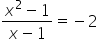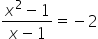Maths-
General
Easy

Question

#What are all values of  that satisfy the equation above?

## -301-3 and -1Hint:

## The correct answer is: -3

### Explanation:We have given an equationWe have to find the value of x , that satisfies the given, equation.We will first do cross multiplication and then solve the equation.Step 1 of 1:The given expression isOn cross multiplication, we will getStep 2 of 2:Using splitting the middle term we will solve the above quadratic equationx= 1 is not possible because it makes the given expressionundefinedSo, x=- 3  is the solution.Hence, Option A is correct. .#### With Turito Foundation.#### Get an Expert Advice From Turito.